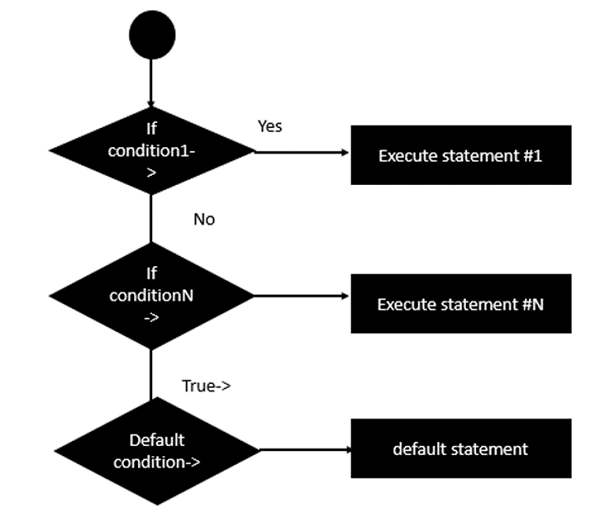# Erlang - Multiple Expression

The if expression also allows for multiple expressions to be evaluated at once. The general form of this statement in Erlang is shown in the following program −

## Syntax

```if
condition1 ->
statement#1;
condition2 ->
statement#2;
conditionN ->
statement#N;
true ->
defaultstatement
end.
```

In Erlang, the condition is an expression which evaluates to either true or false. If the condition is true, then statement#1 will be executed. Else the next condition is evaluated and so on and so forth. If nothing evaluates to true then the defaultstatement is evaluated.

The following image is a general diagrammatic representation of the above given statement.The following program is an example of a simple if expression in Erlang −

## Example

```-module(helloworld).
-export([start/0]).

start() ->
A = 5,
B = 6,
if
A == B ->
io:fwrite("A is equal to B");
A < B ->
io:fwrite("A is less than B");
true ->
io:fwrite("False")
end.
```

The following key things need to be noted about the above program −

• The expression being used here is the comparison between the variables A and B.

• The -> operator needs to follow the expression.

• The ; needs to follow statement#1.

• The -> operator needs to follow the true expression

• The statement ‘end’ needs to there to signify the end of the if block.

The output of the above program will be −

## Output

```A is less than B
```
erlang_decision_making.htm